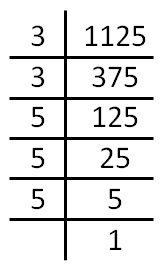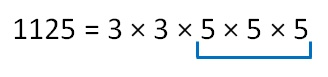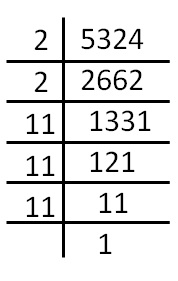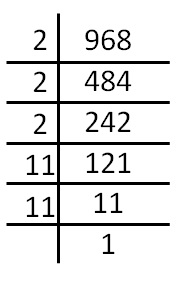Smallest number divided to get a perfect cube

Let’s do this by examples

## Finds the smallest number divided to 1125 to get a perfect cubeWe see that,Since 3 does not occur triplets,

we divide by 3 × 3 to make a triplet

So, our number becomes

1125 × 1/ 3 × 1/3   = 3 × 3 × 5 × 5 × 5 × 1/3 × 1/3

= 5 × 5 × 5

Now, it becomes a perfect cube.

So, we divide 1125 by 3 × 3

i.e. 9 to make it a perfect cube

## Finds the smallest number divided to 5324 to get a perfect cube.We see that,

5324 = 2 × 2 × 11 × 11 × 11

Here,

2 does not occur in triplets

So, we divide by 2 × 2 to make triplet

So, our number becomes

5324  × 1/2  × 1/2 = 2 × 2 × 11 × 11 × 11 × 1/2   × 1/2

= 11 × 11 × 11

Now, it becomes a perfect cube.

So, we divide 5324 by 2 × 2

i.e. to make it a perfect cube

## Finds the smallest number divided to 968 to get a perfect cube.We see that,

968 = 2 × 2 × 2 × 11 × 11

Here,

11 does not occur in triplets

So, we divide by 11 × 11 to make triplet

So, our number becomes

968 × 1/11 × 1/ 11 = 2 × 2 × 2 × 11 × 11 × 1/11  × 1/11

= 2 × 2 × 2

Now, it becomes a perfect cube.

Learn in your speed, with individual attention - Teachoo Maths 1-on-1 Class